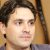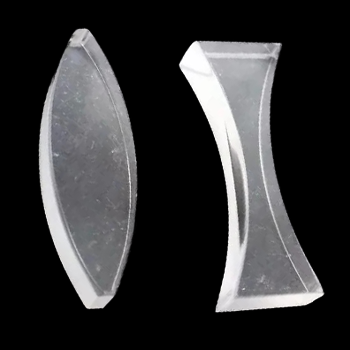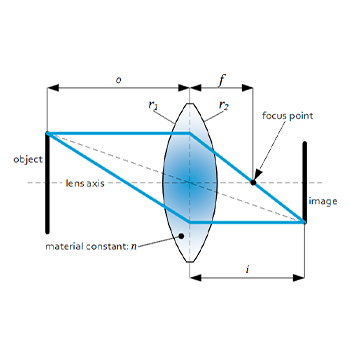# Thin Lens Equation for the MCAT Converging & Diverging ExampleLast Updated On: November 8, 2021

The MCAT is an 8-hour long exam that may determine if you’ll step into that white coat someday.

The crucial part of getting a good score is preparation.

You should know which areas you’ll be tested on the MCAT and the number of questions you’re likely to encounter for each area.

One of the important areas is the light and optics, and thin lens belongs here.

Many students struggle with the thin lens formula, but it doesn’t have to be scary.

I’ve helped thousands of students get ready for the MCAT, and today I’ll use my experience to talk about everything you should know related to the thin lens formula.

## How to Solve Thin Lens Formula on the MCAT

• Light and optics are a medium-yield topic on the MCAT.
• You should know what lenses are and the two different types of lenses.
• Get familiar with the thin lens formula and know how to apply it on the MCAT.

## Lights and Optics on the MCATLight and optics are found on the MCAT because this is important for precision instruments that you see in a lab.

It is a medium-yield topic on the MCAT. Expect that you’ll find it on the MCAT in a biological context, not so much in physics and mathematics.

Light is electromagnetic radiation that the human eye can detect .

The electromagnetic spectrum, i.e., the light waves, includes the waves of all wavelengths.

However, visible light is only a small range of wavelengths. This is the light that makes up the colors that the human eye can see.

## What are Lenses?A lens is a piece of glass used to form an image of an object by focusing light from the object.

Lenses are optical devices, and they have to be curved.

Like mirrors, they change the direction of light rays that go through them — this change of direction results in image formation.

There are several types of lenses.

### Convex and Concave LensesLenses with thicker centers than edges are convex, while lenses with thicker edges than centers are concave .

The light that goes through a convex lens is focused on a point on the other side of the lens. It is what we call the focal point.

The image that’s formed by a convex lens is real. The image distance is positive, while the object distance is negative.

On the other hand, concave lenses don’t condense light beams, they diverge them, and the focal point is in front of the lens.

The focal point is the point on the axis of the incoming light.

For a concave lens, the virtual image is on the same side of the lens as the object. Because of this, the image distance is negative.

The strength of a lens is measured in diopters, and it measures how short the focal length is.

P=1f

p=lens strength

f=focal length

## Thin LensesHere’s the thin lens formula:

1f=1dₒ+ 1dᵢ

The trick is to know when these are positive and when they are negative.

f is the focal length. When you have a thin lens, there’s a focal point on both sides.

The focal length is calculated as the distance from the center of the lens to the focal point (on either side).

To know if the focal length is positive or negative, you have to check what kind of lens you have.

If it’s a convex or a converging lens, the focal length is always positive.

If you have a concave or a diverging lens, it’ll also have two focal points. For a concave or diverging lens, the focal length is always negative.

“It doesn’t matter if it’s a real or a virtual image. Always check what kind of lens you have. That’ll tell you if you should plug in a positive or negative focal length.” Khan Academy

dₒ is the object distance, and it refers to the distance of an object from the center to the lens.

dᵢ is the image distance, and it refers to the distance from the image to the lens.

hₒ is the height of the object.

hᵢ is the height of the image.

Images that are upright in relation to the object have positive heights. Images that are inverted in relation to the object have negative heights.

M is the magnification — ratio of image to object height.

The magnification is related to image and object distance and height with this relation:

hᵢhₒ= -dᵢdₒ=m

Both of these equations are referred to as thin lens equations.

Related Articles:

## Thin Lens Formula MCAT: Final Thoughts

I’ve covered everything you should know about the thin lens formula on the MCAT.

While lights and optics is a medium-yield topic, don’t completely disregard it as you’re likely to get some questions related to them as well.

As for the thin lens formula, keep the explanation above on hand.

I always recommend going through as many practice questions as possible until you can do them without needing extra help.

Make sure to study this guide in detail, and you won’t have any issues with thin lenses on your MCAT exam.

References:

1. https://www.britannica.com/science/light
2. https://global.canon/en/technology/s_labo/light/003/02.html